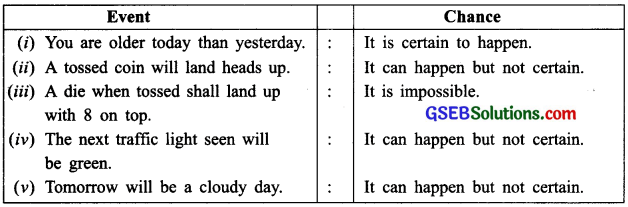# GSEB Solutions Class 7 Maths Chapter 3 Data Handling Ex 3.4

Gujarat Board GSEB Textbook Solutions Class 7 Maths Chapter 3 Data Handling Ex 3.4 Textbook Questions and Answers.

## Gujarat Board Textbook Solutions Class 7 Maths Chapter 3 Data Handling Ex 3.4Question 1.
Tell whether the following is certain to happen, impossible, an happen but not certain.
(i) You are older today than yesterday.
(ii) A tossed coin will land heads up.
(iii) A die when tossed shall land up with 8 on top.
(iv) The next traffic light seen will be green
(v) Tomorrow will be a cloudy day.
Solution:Question 2.
There are 6 marbles in a box with numbers from 1 to 6 marked on each of them.
(i) What is the probability of drawing a marble with number 2?
(ii) What is the probability of drawing a marble with number 5?
Solution:
Total number of marbles = 6
∴ Total number of possible outcomes = 6
(i) Probability of drawing marble with number 2,
p(2) = $$\frac { 1 }{ 6 }$$

(ii) Probability of drawing marble with number 5,
p(5) = $$\frac { 1 }{ 6 }$$Question 3.
A coin is flipped to decide which team starts the game. What is the probability that your team will start?
Solution:
Since there are two faces of a coin, head (H) and tail (T).
When a coin is tossed, then either a ‘H’ or a ‘T’ conies.
∴ Successfully achieved outcome = 1
Total number of possible outcomes = 2
[ ∵ Head + Tail =1 + 1= 2 ]
∴ Required probability = $$\frac { 1 }{ 2 }$$.